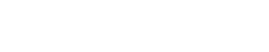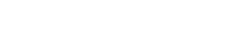# Concrete Mix Design as per IS 10262-2009 – Procedure and CalculationsReading time: 1 minute

Procedure for concrete mix design calculation as per IS 10262-2009 based on strength and durability, workability, economy is discussed in this article. To produce concrete of required strength and properties, selection of ingredients and their quantity is to be found which is called concrete mix design. Proper mix design will solve every problem arises in concrete while placing or curing etc.. The mix design also helps to produce economical concrete. Generally, cement is more costly than other ingredients of concrete. So, quantity and quality of cement is designed by proper mix design concept. In this article we are going to discuss about the concrete mix design concept as per IS 10262-2009.Concrete mix design concept is majorly depending upon the following
• Strength and durability
• Workability
• Economy
• specifications

## Concrete Mix Design Procedure as per is 10262 – 2009

Procedure for concrete mix design requires following step by step process:
1. Calculation of target strength of concrete
2. Selection of water-cement ratio
3. Determination of aggregate air content
4. Selection of water content for concrete
5. Selection of cement content for concrete
6. Calculation of aggregate ratio
7. Calculation of aggregate content for concrete
8. Trial mixes for testing concrete mix design strength

### Step 1: Calculation of Target Strength of Concrete

Target strength is denoted by ft which is obtained by characteristic compressive strength of concrete at 28 days (fck) and value of standard deviation (s)

ft = fck + 1.65 s

Standard deviation can be taken from below table
 Grade of concrete Standard deviation (N/mm2) M10 3.5 M15 3.5 M20 4.0 M25 4.0 M30 5.0 M35 5.0 M40 5.0 M45 5.0 M50 5.0

### Step 2: Selection of Water-Cement Ratio

Ratio of the weight of water to weight of cement in the concrete mix is water-cement ratio. It is the important consideration in concrete mix design to make the concrete workable. Water cement ratio is selected from the below curve for 28 days characteristic compressive strength of concrete.Fig: Selection of Water-Cement Ratio for Concrete Mix Design

Similarly, we can determine the water-cement ration from the 7-day concrete strength, the curves are divided on the basis of strength from water cement ratio is decided. Which is observed from the below graph.### Step 3: Determination of Aggregate Air content

Air content in the concrete mix is determined by the nominal maximum size of aggregate used. Below table will give the entrapped air content in percentage of volume of concrete.
 Nominal maximum size of aggregate Air content (% of volume of concrete) 10mm 5% 20mm 2% 40mm 1%

### Step 4: Selection of Water Content for Concrete

Select the water content which is useful to get required workability with the help of nominal maximum size of aggregate as given in below table. The table given below is used when only angular shaped aggregates are used in concrete as well as the slump should be 25 to 50mm.
 Nominal maximum size of aggregate Maximum water content 10mm 208 20mm 186 40mm 165
If the shape of aggregate or slump value is differing from above, then some adjustments are required as follows.
 Condition Adjustment Sub angular aggregate Reduce the selected value by 10% Gravel with crushed stone Reduce the selected value by 20kg Rounded gravel Reduce the selected value by 25kg Using plasticizer Decrease the selected value by 5-10% Using superplasticizer Decrease the selected value by 20-30% For every increment of 25mm slump Increase the selected value by 3%

### Step 5: Selection of Cement Content for Concrete

Water – cement ratio is determined in step2 and quantity of water is determined in step -4. So, we can easily calculate the quantity of cement from these two conditions. But, the value obtained should satisfy the minimum conditions as given in the below table. The greater of the two values is decided as quantity of cement content.

#### Cement Content for Plain Cement Concrete

 Exposure Plain Cement Concrete (P.C.C) Minimum Cement Content Kg/m3 Max Free Water – Cement Ratio Minimum Grade of Concrete Mild 220 0.6 - Moderate 240 0.6 M15 Severe 250 0.5 M20 Very severe 260 0.45 M20 Extreme 280 0.4 M25

#### Cement Content for Reinforced Concrete

 Exposure Reinforced Cement Concrete (RCC) Minimum Cement Content Kg/m3 Max Free Water –Cement Ratio Minimum Grade of Concrete Mild 300 0.55 M20 Moderate 300 0.5 M25 Severe 320 0.45 M30 Very severe 340 0.45 M35 Extreme 360 0.4 M40

### Step 6: Calculation of Aggregate Ratio

For the given nominal maximum size of aggregate, we can calculate the ratio of volumes of coarse aggregate and volume of total aggregates for different zones of fine aggregates from the below table.
 Nominal maximum size of aggregate Ratio of volume of coarse aggregate and volume of total aggregate for different zones of fine aggregate Zone – 1 Zone – 2 Zone – 3 Zone – 4 10mm 0.44 0.46 0.48 0.50 20mm 0.6 0.62 0.64 0.66 40mm 0.69 0.71 0.73 0.75

### Step 7: Calculation of Aggregate Content for Concrete

We already determine the coarse aggregate volume ratio in the total aggregate volume. So, it is very easy that, 1 – volume of coarse aggregate will give the volume of fine aggregate. Alternatively, there are some formulae to find the volume of fine and coarse aggregates as follows. Mass of fine aggregate is calculated from below formulaSimilarly, mass of coarse aggregate is calculated from below formula.Where, V = volume of concrete W = water content C = cement content Gc = sp. Gravity of cement P = aggregate ration obtained in step6 F.A & C.A = masses of fine and coarse aggregates Gf & Gca = sp. Gravities of fine and coarse aggregates.

### Step 8: Trial Mixes for Testing Concrete Mix Design Strength

Based on the values obtained above, conduct a trail test by making at least 3 cubes of 150mm size as per above standards. Test that cubes and verify whether the required strength is gained or not. If not, redesign the mix with proper adjustments until required strength of cube occurs. Read More: